# What is an Ohmmeter ?The ohmmeter is one of the important electrical tools. However, not everyone knows this device well. Let’s find out more details about Ohmmeter in this article.

What is an Ohmmeter?

An Ohmmeter is an electrical tool used to measure the resistance of a circuit or matter block. The result of resistance measurement in units of resistance is Ohm or the symbol Ω. The unit is named after German physicist Georg Simon Ohm.

In fact, the Ohmmeter’s measurement capability is divided into different bands:

• Micro ohmmeter: perform low resistance measurements, like those of a metal.
• Common Ohmmeter: measure common resistors.
• Mega Ohmmeter (whose registration name is Megger): measures large values of resistance, such as the insulation of a dielectric block.

Operations of an Ohmmeter

The traditional design of the ohmmeter included a small battery that provided a voltage for resistance. A galvanometer measures the current through the resistor and, according to Ohm’s law, deduces the value of the resistance. Along with an ammeter and voltmeter, the ammeter is also used as a function of a multimeter.Ohmmeter symbolOhmmeter face

Serial Ohmmeter

The serial ohmmeter with resistance should be measured in series with the indicator structure (I.S). This type of meter is usually used to measure resistance values Rx sized Ω or more.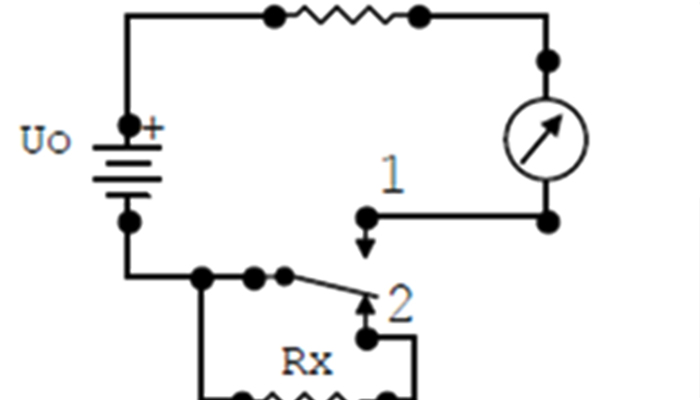the serial Ohmmeter

Rp is the I.S protection extra resistance, ensuring that when Rx = 0 current flows through the largest measuring device (graduated scale)

The internal resistance of Ohmmeter: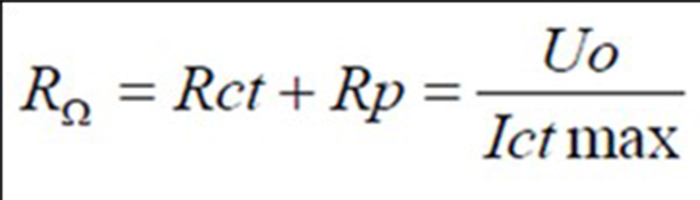Characteristics of a serial ohmmeter

• The Ohmmeter scale follows the opposite direction of the ammeter and voltmeter.
• Ohmmeter readings depend on the internal battery, so to reduce this error, we add potentiometer Rm.

For accurate results even though the battery is low, each time using the Ohmmeter we perform dynamic zero adjustments/ ADJ: input short circuit (for Rx = 0 by shorting the two ends of the probe together), Turn the control knob of Rm so that the needle points zero on the scale.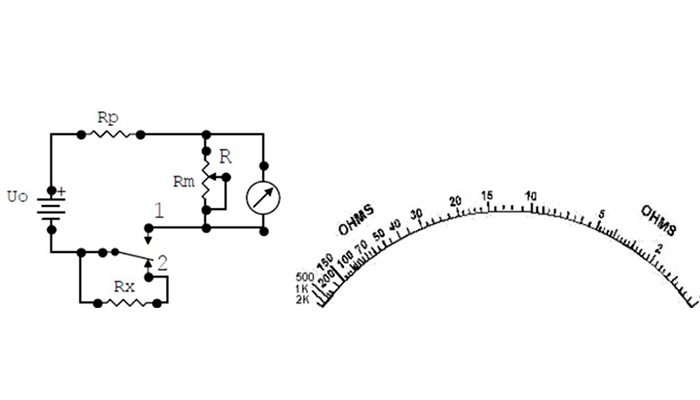The serial Ohmmeter Diagram uses Rm potentiometer

What is parallel Ohmmeter?

The parallel Ohmmeter installs the Rx resistor which needs to measure parallel to I.S. This type of Ohmmeter is usually used to measure small Rx resistance values.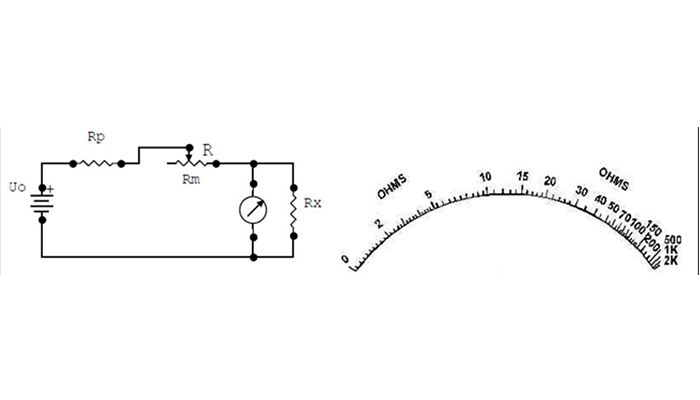The Parallel Ohmmeter Diagram uses Rm potentiometer

Features of the parallel Ohmmeter

• The Ohmmeter scale is parallel in the same direction as the ammeter and voltmeter scale and is non-linear.
• To ensure accurate results, add potentiometer Rm.

The general concept of resistance measurement

There are 2 methods of measuring resistance parameters of the circuit: direct and indirect measurement.

Indirect measurement.

Use an ammeter and voltmeter to measure current and pressure. Then from the equations, the law specifies resistor parameters.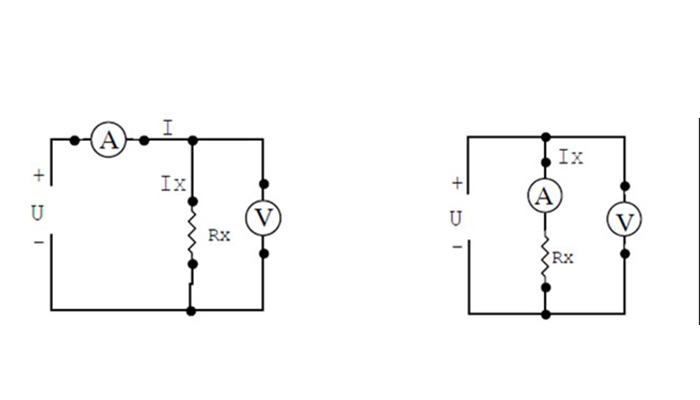Diagram of installing the resistance measurement ammeter and voltmeter based on Ohm’s law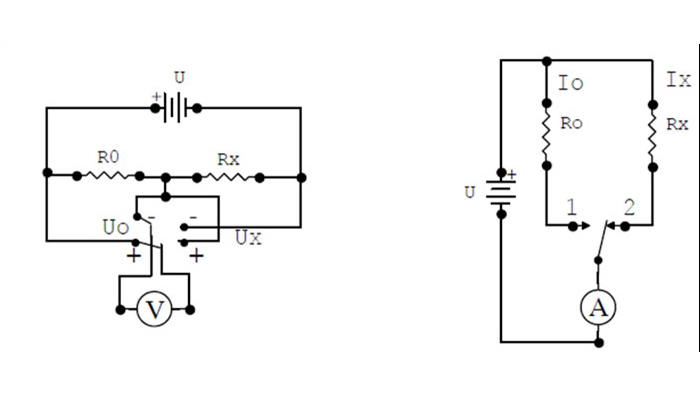Diagram with an ammeter or voltmeter with resistive measurement samples

Direct measurement

Use devices that directly determine the parameter to be measured, called the Ohmmet or the Ohmometer.

Hopefully, the information on Ohmmeter has helped you to have more knowledge about this tool. See you in the next posts.

Source: Wikipedia